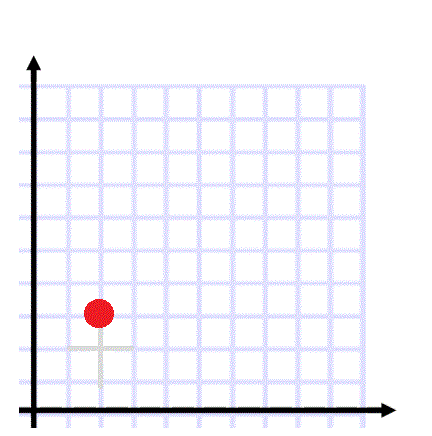# Creating Parallelograms

Directions: Using the digits 1 to 9 at most one time each, fill in the blanks to create a paralelogram from the vertex (2,3): (__,__), (__,__), and (__,__)### Hint

What properties make a parallelogram?
What information do you need to know about the sides?

Answers can vary, one solution is (1,9),(7,2)and(6,8)

Source: Bryan Anderson

## Similar Triangles 2

Directions: Using the digits 0 to 9 at most one time each, create two similar …

### One comment

1.Using the digits 1 to 9 at most one time each, fill in the blanks to create a square from the vertex (2,3): (__,__), (__,__), and (__,__)
Is it possible?